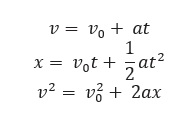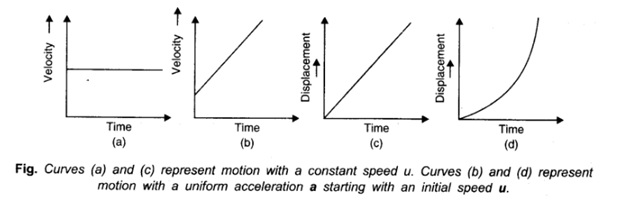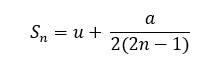For objects in uniformly accelerated rectilinear motion, the five quantities, displacement x, the time took t, initial velocity v0, final velocity v and acceleration a are related by a set of simple equations called kinematic equations of motion :if the position of the object at time t = 0 is 0. If the particle starts at x = x0 , x in above equations is replaced by (x- x0).

## Kinematical Graphs

The ‘displacement-time’ and the ‘velocity-time’ graphs of a particle are often used to provide us with a visual representation of the motion of a particle. The ‘shape’ of the graphs depends on the initial ‘co-ordinates’ and the ‘nature’ of the acceleration of the particle (Fig.)## Equations of Uniformly Accelerated Motion

If a body starts with velocity (u) and after time t its velocity changes to v, if the uniform acceleration is a and the distance traveled in time t in s, then the following relations are obtained, which are called equations of uniformly accelerated motion.

1. v = u + at
2. s = ut + at2
3. v2 = u2 + 2as

Distance traveled in nth secondIf a body moves with uniform acceleration and velocity changes from u to v in a time interval, then the velocity at the midpoint of its path

√u2 + v2/ 2

## Motion under Gravity

If an object is falling freely (u = 0) under gravity, then equations of motion

1. v = u + gt
2. h =ut +gt2
3. v2 = u2 + 2gh

Note: If an object is thrown upward then g is replaced by – g in above three equations. It thus follows that

###Maximum height reached by the body:A ball is dropped from a building of height h and it reaches after t seconds on earth. From the same building if two balls are thrown (one upwards and other downwards) with the same velocity u and they reach the earth surface after t, and t2 seconds respectively, thenWhen a body is dropped freely from the top of the tower and another body is projected horizontally from the same point, both will reach the ground at the same time.